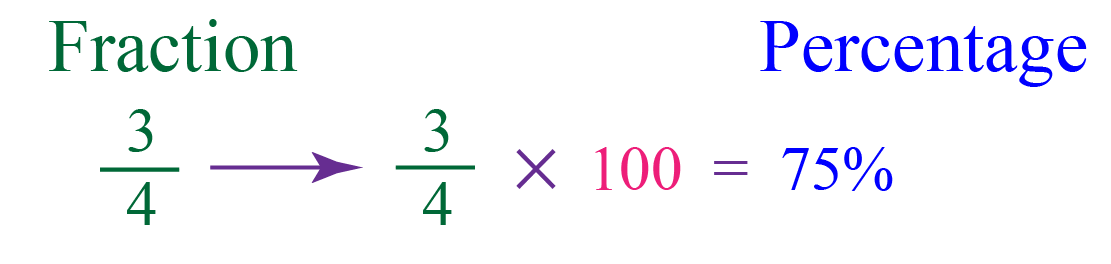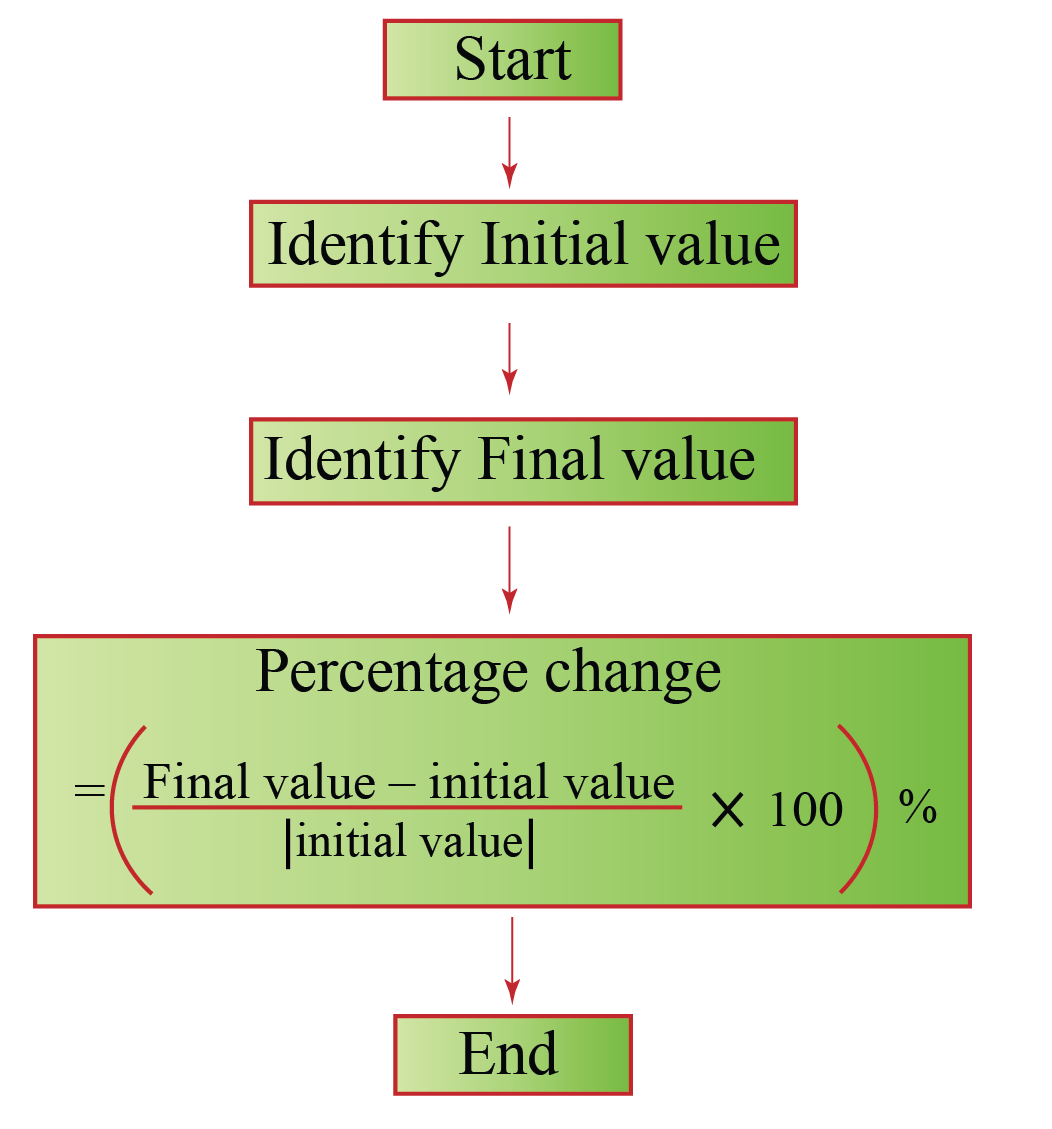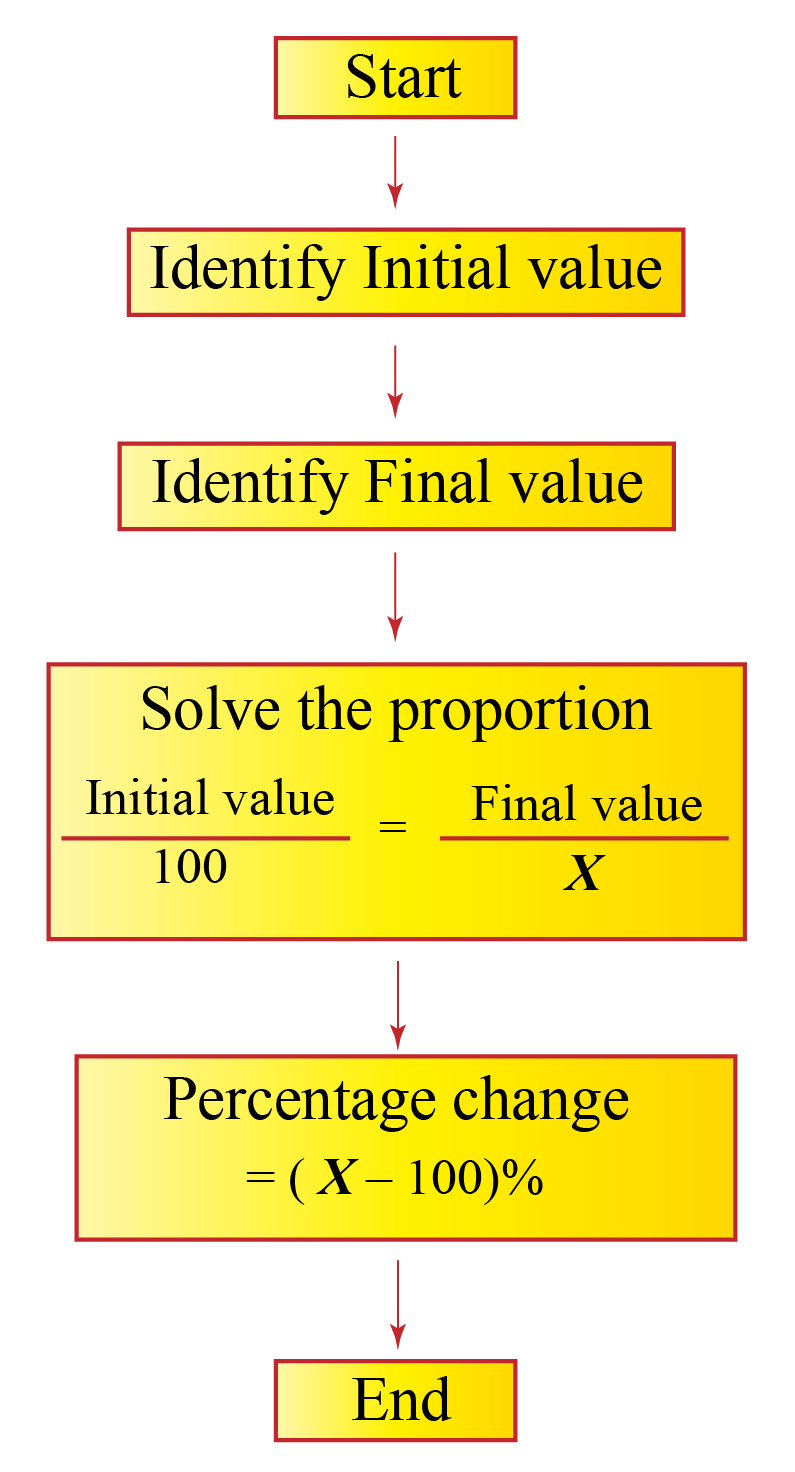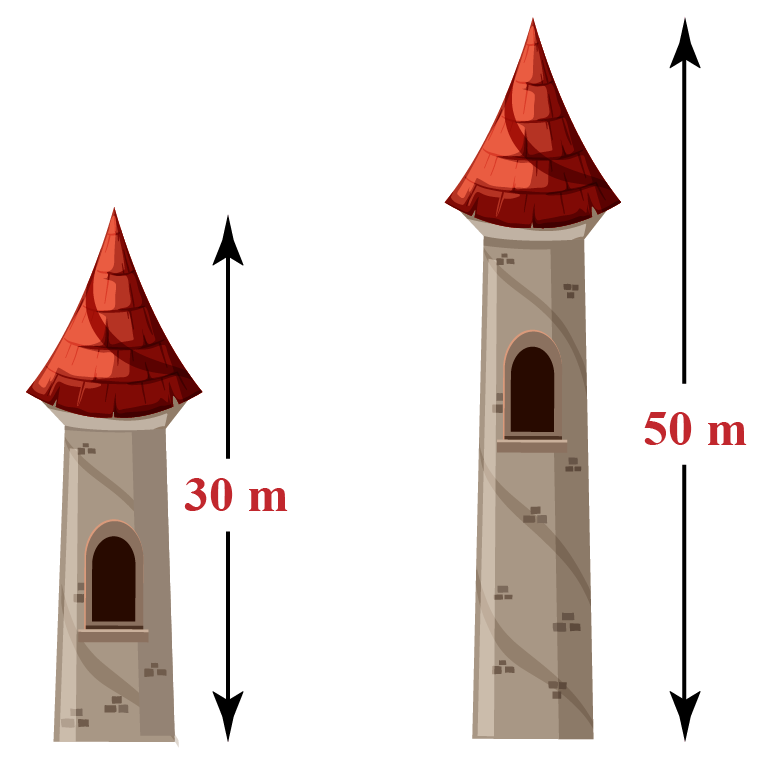# Percentage Change

 1 Understanding Percentage Change 2 Definition of Percentage Change 3 Formula of Percentage Change 4 How to calculate Percentage Change Step by Step? 5 Important Notes on Percentage Change 6 Percentage Change Calculator 7 Solved Examples on Percentage Change 8 Thinking out of the Box! 9 Practice Questions on Percentage Change 11 Maths Olympiad Sample Papers 10 Frequently Asked Questions (FAQs)

We at Cuemath believe that Math is a life skill. Our Math Experts focus on the “Why” behind the “What.” Students can explore from a huge range of interactive worksheets, visuals, simulations, practice tests, and more to understand a concept in depth.

Book a FREE trial class today! and experience Cuemath’s LIVE Online Class with your child.

## Understanding Percent Change

Robert started a business with an initial investment of Rs. 30,000 and in one year, it grew to Rs. 70,000.

Michael started a business at the same time as Robert with an initial investment of Rs. 25,000 and in one year, it grew to Rs. 65,000

Growth
(Final Value - Initial Value)

Robert

Rs. 30,000 Rs. 70,000 Rs. 40,000

Michael

Rs. 25,000 Rs. 65,000 Rs. 40,000

Can we say both businesses grew at the same rate because the growth in each business is Rs. 40,000?

No, we can't do so.

The growth rate should always be calculated with respect to the initial value and only then the rates can be compared.

The percent change gives the difference in a quantity with respect to its initial value.

It gives the growth/decay rate.

This helps us in comparing the quantities.

## Definition of Percent Change

The percent change (or) the percent change of a quantity is the ratio of the difference in the quantity to its initial value multiplied by 100

(OR)

The percent change (or) the percent change of a quantity is the percent of its initial value either increased or decreased to get its final value.

As the name suggests, the percent change is the difference in the percent of the quantity.

Generally, to convert a fraction into a percent, we multiply it by 100

For example:In the same way, we multiply the fraction (ratio) of difference in quantity to its initial value by 100 to get the percent change.

The percent change gives the difference in quantity out of 100

## Formula of Percentage Change

The formula of percent difference (or) the equation of percent change is:

 $${\ \left(\dfrac{ \text{Final Value - Initial Value}}{|\text{Initial Value}|} \times 100 \right)}\%$$

## How to calculate Percentage Change Step by Step?

There are two methods to calculate the percent change.

One is using the above formula and the other is using proportions.

Let us solve the following problem using both the methods.

If 64 is increased to 120, find the percent change.

To perform these methods, we need to identify the initial and final values.

\begin{aligned} \text{Initial Value}&=64\\[0.2cm] \text{Final Value}&=120 \end{aligned}

### Method 1

a) Subtract the initial value from the final value

\begin{align} \text{Final Value - Initial Value} &= 120-64 \\ &=56\end{align}

b) Divide this by the absolute value of the initial value

\begin{align}\dfrac{ \text{Final Value - Initial Value}}{|\text{Initial Value}|} &= \dfrac{56}{|64|} \\ &=0.875\end{align}

c) Multiply the result by 100

\begin{align}&\dfrac{ \text{Final Value - Initial Value}}{|\text{Initial Value}|} \times 100\\[0.2cm]& = 0.875\times 100\\[0.2cm]& = 87.5\% \end{align}

Since the answer is positive, it is a percent increase.

 Percent Increase = 87.5%

We can understand this process easily using the following flowchart.### Method 2

In this method, we assume that

• the initial value corresponds to 100%
• the final value corresponds to $$x$$%

Then we can write the following proportion

$\dfrac{64}{100}= \dfrac{120}{x}$ Solve for $$x$$ by cross multiplication. \begin{aligned} 64x &= 12000\\[0.2cm] x &=187.5 \end{aligned}

This is the percent of the final value when compared to the initial value

To find the percent change, we just subtract 100% from this.

\begin{align} \text{Percent change}&= 187.5\% -100\% \\&= 87.5\%\end{align}

Since it is positive, it is a percent increase.

 Percent Increase = 87.5%

We can understand this process easily using the following flowchart.We usually use Method 1 to calculate the percent change.

Help your child score higher with Cuemath’s proprietary FREE Diagnostic Test. Get access to detailed reports, customised learning plans and a FREE counselling session. Attempt the test now.

## Solved Examples

 Example 1

The price of a toy car is decreased from Rs. 20 to Rs. 15 after a discount.

Find the percentage of the discount.Solution:

Initial price of the car = Rs. 20

Final price of the car = Rs. 15

The percentage change (the percentage of discount) is calculated using:

\begin{aligned} &\text{Percentage Change }\\[0.3cm]&= \left( \dfrac{ \text{Final Value - Initial Value}}{ |\text{Initial Value}|} \times 100 \right)\%\\[0.3cm] &= \left( \dfrac{15-20}{|20|} \times 100 \right)\%\\[0.3cm] &= \left( \dfrac{-5}{20} \times 100 \right) \%\\[0.3cm] &= -25 \% \end{aligned}

The negative percentage change indicates percentage decrease.

Discount always leads to a percentage decrease.

Note: If you cross-check the answer, 25% of the initial price which is Rs. 20 is Rs. 5,

and Rs. 20 - Rs. 5 = Rs. 15, which is the price given after the discount.

 $$\therefore$$ The discount percentage = 25%
 Example 2

The length of a tower is increased from 30 m to 50 m.

Find the percentage increase.Solution:

Initial height of the tower = 30 m

Final height of the tower = 50 m

The percentage change is calculated by the formula:

\begin{aligned} &\text{Percentage Change }\\[0.3cm]&=\!\! \left( \dfrac{ \text{Final Height - Initial Height}}{ |\text{Initial Height}|} \!\times\! 100 \right)\!\!\%\\[0.3cm] &=\!\! \left( \dfrac{50-30}{|30|} \times 100 \right)\%\\[0.3cm] &=\!\! \left( \dfrac{20}{30} \times 100 \right) \%\\[0.3cm] &\approx 66.67 \% \end{aligned}

Positive percentage change indicates percentage increase.

 $$\therefore$$ The percentage increase  $$\mathbf{\approx}$$ 66.67%
 Example 3

A number 35 is misread to be 53.

Find the percentage error.

Solution:

Original number = 35

The percentage error (change) is calculated using:

\begin{aligned} &\text{Percentage Change }\\[0.3cm]&=\!\! \left(\! \dfrac{ \text{Misread number - Original number}}{ \text{Original Number}} \!\times\! 100 \!\!\right)\!\!\%\\[0.3cm] &=\!\! \left( \dfrac{53-35}{35} \times 100 \right)\%\\[0.3cm] &=\!\! \left( \dfrac{18}{35} \times 100 \right) \%\\[0.3cm] &\approx 51\% \end{aligned}

 $$\therefore$$ The percentage error $$\mathbf{\approx}$$ 51%Think Tank
1. A number is increased by 20% and then decreased by 20%

Find the net or percentage increase or decrease of the number.

CLUEless in Math? Check out how CUEMATH Teachers will explain Percentage Change to your kid using interactive simulations & worksheets so they never have to memorise anything in Math again!

Explore Cuemath Live, Interactive & Personalised Online Classes to make your kid a Math Expert. Book a FREE trial class today!

## Practice Questions

Here are a few activities for you to practice. Select/Type your answer and click the "Check Answer" button to see the result.

IMO (International Maths Olympiad) is a competitive exam in Mathematics conducted annually for school students. It encourages children to develop their math solving skills from a competition perspective.

## 1. How do you find the percent of change?

The percentage of change is calculated using the formula:

$$\!\!\!\!\!\!\! \left(\!\dfrac{ \text{Final Value - Initial Value}}{|\text{Initial Value}|} \!\!\times\!\! 100 \!\!\right)\!\!\%$$

## 2. Can you calculate percent change of percentages?

Yes, we just calculate it using the formula:

$$\!\!\!\!\!\left(\!\dfrac{ \text{Final Percentage - Initial Percentage}}{\text{Initial Percentage}} \!\!\times\!\! 100 \!\!\right)\!\!\%$$

## 3. What is the meaning of percent of change?

The percent change (or) the percentage change of a quantity is the ratio of the change in the quantity to its initial value multiplied by 100.

## 4. How do I find the percentage of multiple changes?

We need to apply the percentage change formula multiple times as required.

More Important Topics
Numbers
Algebra
Geometry
Measurement
Money
Data
Trigonometry
Calculus